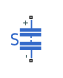# Supercapacitor

Electrochemical double-layer capacitor

• Library:
• Simscape / Electrical / Passive

•## Description

The Supercapacitor block represents an electrochemical double-layer capacitor (ELDC), which is commonly referred to as a supercapacitor or an ultracapacitor. The capacitance values for supercapacitors are orders of magnitude larger than the values for regular capacitors. Supercapacitors can provide bursts of energy because they can charge and discharge rapidly.

You can model any number of supercapacitor cells connected in series or in parallel using a single Supercapacitor block. To do so, set the relevant parameter, that is Number of series cells or Number of parallel cells, to a value larger than `1`. Internally, the block simulates only the equations for a single supercapacitor cell, but it calculates:

• The output voltage according to the number of series-connected cells

• The current according to the number of parallel-connected cells

Calculating the output of a multiple-cell supercapacitor based on the output for a single cell is more efficient than simulating the equations for each cell individually.

The figure shows the equivalent circuit for a single cell in the Supercapacitor block. The circuit is a network of resistors and capacitors that is commonly used to model supercapacitor behavior.Capacitors C1, C2, and C3 have fixed capacitances. The capacitance of capacitor Cv depends on the voltage across it. Resistors R1, R2, and R3 have fixed resistances. The voltage across each individual fixed capacitor in the Supercapacitor block is calculated as

`${V}_{cn}=\frac{v}{{N}_{series}}-{i}_{n}{R}_{n},$`

where:

• v is the voltage across the block.

• Nseries is the number of cells in series.

• n is the branch number. n = [1, 2, 3].

• in is the current through the nth branch.

• Rn is the resistance in the nth branch.

• Vcn is voltage across the capacitor in the nth branch.

The equation for the current through the first branch of the supercapacitor depends on the voltage across the capacitors in the branch. If the capacitors experience a positive voltage, that is

`${V}_{c1}>0,$`

then

`${i}_{1}=\left({C}_{1}+{K}_{v}{V}_{c1}\right)\frac{d{V}_{c1}}{dt},$`

else

`${i}_{1}={C}_{1}\frac{d{V}_{c1}}{dt},$`

where:

• Vc1 is voltage across the capacitors in the first branch.

• C1 is the capacitance of the fixed capacitor in the first branch.

• Kv is the voltage-dependent capacitance gain.

• i1 is the current through the first branch.

For the remaining branches, the current is defined as

`${i}_{n}={C}_{n}\frac{d{V}_{cn}}{dt},$`

where:

• n is the branch number. n =[2, 3].

• Cn is the capacitance of the nth branch.

The total current through the Supercapacitor block is

`$i={N}_{parallel}\left({i}_{1}+{i}_{2}+{i}_{3}+\frac{v}{{R}_{discharge}}\right),$`

where:

• Nparallel is the number of cells in parallel.

• Rdischarge is the self-discharge resistance of the supercapacitor.

• i is the current through the supercapacitor.

## Ports

### Conserving

expand all

Electrical conserving port associated with the positive terminal.

Electrical conserving port associated with the negative terminal.

## Parameters

expand all

Cell Characteristics

Specify the resistances for the fixed resistors in the individual branches of the supercapacitor as an array.

Specify the individual capacitances for the fixed capacitors in the supercapacitor as an array.

Specify the variable capacitance coefficient, Kv, for the voltage-dependent capacitor in the first branch of the supercapacitor. For information on determining the variable capacitance coefficient, see .

Specify the self-discharge resistance of the supercapacitor that is connected between the two terminals.

Configuration

Specify the number of cells in the supercapacitor that are in a series configuration.

Specify the number of cells in the supercapacitor that are in a parallel configuration.

Variables

Use the Variables tab to set the priority and initial target values for the block variables before simulation. For more information, see Set Priority and Initial Target for Block Variables.

 Zubieta, L. and R. Bonert. “Characterization of Double-Layer Capacitors for Power Electronics Applications.” IEEE Transactions on Industry Applications, Vol. 36, No. 1, 2000, pp. 199–205.

 Weddell, A. S., G. V. Merrett, T. J. Kazmierski, and B. M. Al-Hashimi. “Accurate Supercapacitor Modeling for Energy-Harvesting Wireless Sensor Nodes.” IEEE Transactions on Circuits And Systems–II: Express Briefs, Vol. 58, No. 12, 2011, pp. 911–915.